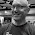## Thursday, March 12, 2020

### Tuning space and write amplification to minimize cost

This uses math to show how to tune space and write amplification to minimize storage costs for an index structure that uses index+log. The result, minimal cost, is true assuming my model is true. But at this point I will only claim that the model is truthy. I look forward to more results in this area from the smart people at DASlab and elsewhere. I wonder if database economics is a good name for this topic.

I explained the index+log index structure here and here and my truthy model assumes that write and space amplification are functions of PctUsed - the percent of available storage that is used by the index structure. The model is:
• Space amplification = 100 / PctUsed
• Write amplification = 100 / (100 - PctUsed)
In what follows I use SpaceAmp and WriteAmp for space and write amplification. When PctUsed is X then the remaining space on the storage device (100-X) is free, not used by anything. The formulas mean that a deployment can trade between SpaceAmp and WriteAmp by adjusting the value of PctUsed. When PctUsed is 80 the values of SpaceAmp and WriteAmp are 1.25 and 5. When PctUsed is 20 the values of SpaceAmp and WriteAmp are 5 and 1.25.

Math

Back in the day hardware was scarce and there was much math in systems papers. While there isn't as much math today the rush to ML means that more people are learning applied math (me included) which is a good thing. I regret not learning enough applied math while in college.

Here I derive a formula for the cost of storage in terms of the database size and the IO required to do compaction (GC, garbage collection) for an index structure that uses the index+log approach. The cost is a function of PctUsed.

Assume:
P = PctUsed
S = index structure size in GB
G = Cost/GB
N = Write rate to index structure in MB/s
f = value between 1 and 2, 1= no storage reads by GC, 2= all GC writes do storage reads first
I = Cost/IOPs for 4KB operations

Cost = Costspace + Costio

# Formulas for the cost of space and IOPs
# 256 converts MB/s into 4KB IOPs

Costspace = S * G * 100 * P-1
Costio = N * 256 * f * I * 100 * (100-P)-1

# Determine where Cost' = 0 to find the minimal cost
Cost' = Costspace+ Costio'

Costspace= -1 * S * G * 100 * P-2
Costio= N * 256 * f * I * 100 * (100-P)-2 * -1 * -1
Cost' = (-1 * S * G * 100 * P-2) + (N * 256 * f * I * 100 * (100-P)-2 )
# And Cost' = 0 when
S * G * 100 * P-2 = N * 256 * f * I * 100 * (100-P)-2
# Skipping a few steps this reduces to
P* ((NfI/SG) - 1) + 200P - 10,000 = 0

# This can be solved by the quadratic equation with a=((NfI/SG) - 1), b=200, c=-10,000

Graphs

Now I solve the equation above to determine the value of PctUsed that minimizes cost with prices from EBS provisioned IOPs. A Google Sheets spreadsheet with the solution is here. For the spreadsheet:
• The graph uses log-scale for the y-axis and the y-axis doesn't start at 0. This makes it easier to see the impact of changing PctUsed, but can also be misleading.
• Cost is computed for PctUsed in (5, 10, 15, ..., 85, 90, 95)
• The minimal value for Cost (quad1) is likely to be between these values
I then solve for 3 cases: N=1, 10 and 100 where N is the write rate to the index structure in MB/s. The minimal cost occurs at PctUsed = 67, 39 and 17 for N = 1, 10 and 100.

For N=1, a low write rate, the minimal cost is at PctUsed=67

For N=10, a moderate write rate, the minimal cost is at PctUsed=39

For N=100, an extreme write rate, the minimal cost is at PctUsed=17

1.Interesting as usual Mark!
Quick question though. I am probably missing some basic maths here, but anyway. When computing Cost'=0 why do you use:

Costspace' = -1 * S * G * 100 * P^(-2)
* Why do you multiply this by -1 at the beginning?

Costio' = N * 256 * f * I * 100 * (100-P)^(-2) * -1 * -1

Thanks!

2.I didn't mention it but Cost' is dCost / dP - will update post for that.
Cost-space is S * G * 100 * P^(-1) and S*G*100 is a constant, call it K
d K*P^(-1) / dP is K * (-1) * P^(-2)
Then replace K by S*G*100 -> S * G * 100 * (-1) * P^(-2)

For Cost-io, N*256*f*I*100 is constant, so d/dP is d (100-P)^(-1) / dP
Derivative of (100-P)^(-1) is (-1) * (100-P)^(-2) * d(100-P)/dP and d(100-P)/dP = (-1)
So we get N*256*f*I*100 * (-1) * (100-P)^(-2) * (-1)

1.One reason for this blog post is to refresh some of the math I learned last year and have started to forget